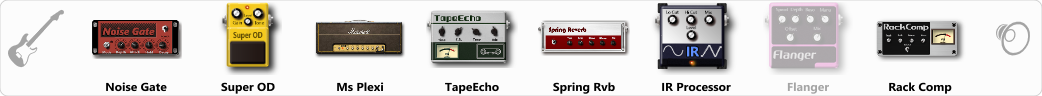# Lenny Kravitz

Discussion in 'ToneLib-GFX presets' started by BlackTears, Feb 5, 2022.

1. Lenny Kravitz

Preset name: Lenny Kravitz

Effects chain:Effect: "Noise Gate" (Dynamics / Filter), active - "yes"
"Detect" = Gtr In
"Mode" = Manual
"Depth" = 50
"Threshold" = 50
"Attack" = 0
"Hold" = 5
"Decay" = 250

Effect: "Super OD" (Overdrive / Distortion), active - "yes"
"Drive" = 0
"Tone" = 100
"Level" = 100

Effect: "Ms Plexi" (Amp simulators), active - "yes"
"Gain" = 82
"Bass" = 70
"Middle" = 66
"Treble" = 72
"Presence" = 68
"Master" = 50
"Output" = 50
"Level (dB)" = 0

Effect: "TapeEcho" (Delay), active - "yes"
"Time" = 214
"Feedback" = 38
"Tone" = 65
"LoDamp" = 19
"Mix" = 70

Effect: "Spring Rvb" (Reverb), active - "yes"
"Time" = 10.0
"PreDelay" = 40
"LoDamp" = 100
"HiDamp" = 100
"Mix" = 50

Effect: "IR Processor" (Dynamics / Filter), active - "yes"
"IR" = battery_stereo_IR
"Low Cut (Hz)" = 0
"Hi Cut (kHz)" = 20.0
"Mix" = 100
"Level (dB)" = 0

Effect: "Flanger" (Modulation / Sfx), active - "no"
"Speed" = 0.8
"Depth" = 71
"Reso" = 35
"Center" = 7.7
"Offset" = 0.0
"Mix" = 75
"Reset" = On

Effect: "Rack Comp" (Dynamics / Filter), active - "yes"
"Threshold (dB)" = -20
"Ratio" = 5
"Attack" = Fast
"Release (ms)" = 250
"Knee" = 25
"Level (dB)" = 7

Note: You will need to download and install the ToneLib-GFX software to use the preset.

#### Attached Files:

• ###### Lenny_Kravitz.tlgfx
File size:
1.7 KB
Views:
1,800
Anrew likes this.